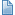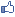# Broj PI na 1000 decimala

 [es] :: Vodič za učenje :: Seminarski radovi :: Broj PI na 1000 decimala [ Pregleda: 3486 | Odgovora: 3 ] > FB > Twit Postavi temu Odgovori

Autor

 Pretraga teme: Traži Markiranje Štampanje RSS

BlackSnake
Zenica, BA

Član broj: 11245
Poruke: 219
*.telecom.ba.

ProfilBroj PI na 1000 decimala 13.06.2003. u 15:50 - pre 239 meseci
Pozdrav svima,
Ja sam već jednu poruku poslao, ali veze nemam gdje je završila, pa ću ponoviti.
Ovo je u stvari poziv u pomoć.
Student sam I godine Elektrotehnike imam zadatak da u kratkom roku uradim seminarski iz prog.jezika C++. Tema rada je:
"Izračunati broj PI na 1000 decimala".
Ja tek treba da startam sa ovim programskim jezikom, pa molim nekog od programera (kojima ovo vjerovatno neće biti problem), da mi izradi ovaj program, a ja ću ga uporedo sa učenjem nastojati razumjeti.

Hvala.
When you think everithing happens to you is so bad ....
remember that always could be even worse.
 Odgovor na temu

ventura

Član broj: 32
Poruke: 7781
*.verat.net

+6455ProfilRe: Broj PI na 1000 decimala 13.06.2003. u 15:54 - pre 239 meseci

Code:
/* +++Date last modified: 05-Jul-1997 */
#include <stdio.h>
#include <stdlib.h>
#include <math.h>
#include <time.h>
#include "snipmath.h"
#ifdef __TURBOC__
typedef short int Indexer;
#else
typedef long int Indexer;
#endif
typedef short int Short;
typedef long int Long;
short *a, *b, *c, *Work1, *MulWork, *Work2, *Work3;
int SIZE;
/*
** Work1 is explicitly used in Reciprocal() and RSqrt()
** Work1 is implicitly used in Divide() and Sqrt()
** Work2 is explicitly used in Divide() and Sqrt()
** Work3 is used only in the AGM and holds the previous reciprocal
** square root, allowing us to save some time in RSqrt()
*/
void Copy(Short *a, Short *b, Indexer Len)

{
while (Len--) a[Len] = b[Len];
}
/*
** this rounds and copies a 2x mul result into a normal result
** Our number format will never have more than one unit of integer,
** and after a mul, we have two, so we need to fix that.
*/
void Round2x(Short *a, Short *b, Indexer Len)

{
Indexer x;
short carry;
carry = 0;
if (b[Len+1] >= 5000)
carry = 1;
for (x = Len; x > 0; x--)

{
carry += b[x];
a[x-1] = carry % 10000;
carry /= 10000;
}
}
void DivBy2(Short *n, Indexer Len)

{
Indexer x;
long temp;
temp = 0;
for (x = 0; x < Len; x++)

{
temp = (Long)n[x]+temp*10000;
n[x] = (Short)(temp/2);
temp = temp%2;
}
}
void DoCarries(Short *limit, Short *cur, Short carry)

{
long temp;
while ((cur >= limit) && (carry != 0))

{
temp = *cur+carry;
carry = 0;
if (temp >= 10000)

{
carry = 1;
temp -= 10000;
}
*cur = temp;
--cur;
}
}
void DoBorrows(Short *limit, Short *cur, Short borrow)

{
long temp;
while ((cur >= limit) && (borrow != 0))

{
temp = *cur-borrow;
borrow = 0;
if (temp < 0)

{
borrow = 1;
temp += 10000;
}
*cur = temp;
--cur;
};
}
void PrintShort2(char *str, Short *num, Indexer Len)

{
Indexer x;
printf("%s ", str);
printf("%u.", num);
for (x = 1; x < Len; x++)
printf("%04u", num[x]);
printf("\n");
}
void PrintShort(char *str, Short *num, Indexer Len)

{
Indexer x;
int printed = 0;
printf("%s ", str);
printf("%u.\n", num);
for (x = 1; x < Len; x++)

{
printf("%02d", num[x]/100);
printed += 2;
if ((printed % 1000) == 0)

{
printf("\n\n\n\n");
printed = 0;
}
else if ((printed % 50) == 0)

{
printf("\n");
}
else if ((printed % 10) == 0)

{
printf(" ");
}
printf("%02d", num[x] % 100);
printed += 2;
if ((printed % 1000) == 0)

{
printf("\n\n\n\n");
printed = 0;
}
else if ((printed % 50) == 0)

{
printf("\n");
}
else if ((printed % 10) == 0)

{
printf(" ");
}
}
printf("\n");
}
/* sum = a + b */
short Add(Short *sum, Short *a, Short *b, Indexer Len)

{
long s, carry;
Indexer x;
carry = 0;
for (x = Len - 1; x >= 0; x--)

{
s = (Long)a[x] + (Long)b[x] + carry;
sum[x] = (Short)(s%10000);
carry = s/10000;
}
return carry;
}
/* dif = a-b */
short Sub(Short *dif, Short *a, Short *b, Indexer Len)

{
long d, borrow;
Indexer x;
borrow = 0;
for (x = Len - 1; x >= 0; x--)

{
d = (Long)a[x] - (Long)b[x] - borrow;
borrow = 0;
if (d < 0)

{
borrow = 1;
d += 10000;
}
dif[x] = (Short)d;
}
return borrow;
}
void Negate(Short *num, Indexer Len)

{
Indexer x;Long d, borrow;
borrow = 0;
for (x = Len - 1; x >= 0; x--)

{
d = 0 - num[x] - borrow;
borrow = 0;
if (d < 0)

{
borrow = 1;
d += 10000;
}
num[x] = (Short)d;
}
}
/* prod = a*b. prod should be twice normal length */
void Mul(Short *prod, Short *a, Short *b, Indexer Len)

{
long p;
Indexer ia, ib, ip;
if ((prod == a) || (b == prod))

{
printf("MUL product can't be one of the other arguments\n");
exit(1);
}
for (ip = 0; ip < Len * 2; ip++)
prod[ip] = 0;
for (ib = Len - 1; ib >= 0; ib--)

{
if (b[ib] == 0)
continue;
ip = ib + Len;
p = 0;
for (ia = Len - 1; ia >= 0; ia--)

{
p = (Long)a[ia]*(Long)b[ib] + p + prod[ip];
prod[ip] = p%10000;
p = p/10000;
ip--;
}
while ((p) && (ip >= 0))

{
p += prod[ip];
prod[ip] = p%10000;
p = p/10000;
ip--;
}
}
}
/*
** this is based on the simple O(n^1.585) method, although my
** growth seems to be closer to O(n^1.7)
**
** It's fairly simple. a*b is: a2b2(B^2+B)+(a2-a1)(b1-b2)B+a1b1(B+1)
**
** for a = 4711 and b = 6397, a2 = 47 a1 = 11 b2 = 63 b1 = 97 Base = 100
**
** if we did that the normal way, we'd do
** a2b2 = 47*63 = 2961
** a2b1 = 47*97 = 4559
** a1b2 = 11*63 = 693
** a1b1 = 11*97 = 1067
**
** 29 61
**45 59
** 6 93
**10 67
** -----------
** 30 13 62 67
**
** Or, we'd need N*N multiplications.
**
** With the 'fractal' method, we compute:
** a2b2 = 47*63 = 2961
** (a2-b1)(b1-b2) = (47-11)(97-63) = 36*34 = 1224
** a1b1 = 11*97 = 1067
**
** 29 61
**29 61
**12 24
**10 67
**10 67
** -----------
** 30 13 62 67
**
** We need only 3 multiplications, plus a few additions. And of course,
** additions are a lot simpler and faster than multiplications, so we
**
** The way it is written requires that the size to be a power of two.
** That's why the program itself requires the size to be a power of two.
** There is no actual requirement in the method, it's just how I did it
** because I would be working close to powers of two anyway, and it was
** easier.
*/
void FastMul(Short *prod, Short *a, Short *b, Indexer Len)

{
Indexer x, HLen;
int SignA, SignB;
short *offset;
short *NextProd;
/*
** this is the threshold where it's faster to do it the ordinary way
** On my computer, it's 16. Yours may be different.
*/
if (Len <= 16 )

{
Mul(prod, a, b, Len);
return;
}
HLen = Len/2;
NextProd = prod + 2*Len;
SignA = Sub(prod, a, a + HLen, HLen);
if (SignA)

{
SignA = 1;
Negate(prod, HLen);
}
SignB = Sub(prod + HLen, b + HLen, b, HLen);
if (SignB)

{
SignB = 1;
Negate(prod + HLen, HLen);
}
FastMul(NextProd, prod, prod + HLen, HLen);
for (x = 0; x < 2 * Len; x++)
prod[x] = 0;
offset = prod + HLen;
if (SignA == SignB)
DoCarries(prod, offset - 1, Add(offset, offset, NextProd, Len));
else DoBorrows(prod, offset - 1, Sub(offset, offset, NextProd, Len));
FastMul(NextProd, a, b, HLen);
offset = prod + HLen;
DoCarries(prod, offset - 1, Add(offset, offset, NextProd, Len));
FastMul(NextProd, a + HLen, b + HLen, HLen);
offset = prod + HLen;
DoCarries(prod, offset - 1, Add(offset, offset, NextProd, Len));
offset = prod + Len;
DoCarries(prod, offset - 1, Add(offset, offset, NextProd, Len));
}
/*
** Compute the reciprocal of 'a'.
** x[k+1] = x[k]*(2-a*x[k])
*/
void Reciprocal(Short *r, Short *n, Indexer Len)

{
Indexer x, SubLen;
int iter;
double t;
/* Estimate our first reciprocal */
for (x = 0; x < Len; x++)
r[x] = 0;
t = n + n*1.0e-4 + n*1.0e-8;
if (t == 0.0)
t = 1;
t = 1.0/t;
r = t;
t = (t - floor(t))*10000.0;
r = t;
t = (t - floor(t))*10000.0;
r = t;
iter = log(SIZE)/log(2.0) + 1;
SubLen = 1;
while (iter--)

{
SubLen *= 2;
if (SubLen > SIZE)
SubLen = SIZE;
FastMul(MulWork, n, r, SubLen);
Round2x(Work1, MulWork, SubLen);
MulWork = 2;
for (x = 1; x < Len; x++)
MulWork[x] = 0;
Sub(Work1, MulWork, Work1, SubLen);
FastMul(MulWork, r, Work1, SubLen);
Round2x(r, MulWork, SubLen);
}
}
/*
** Computes the reciprocal of the square root of 'a'
** y[k+1] = y[k]*(3-a*y[k]^2)/2
**
**
** We can save quite a bit of time by using part of our previous
** results! Since the number is converging onto a specific value,
** at least part of our previous result will be correct, so we
** can skip a bit of work.
*/
void RSqrt(Short *r, Short *n, Indexer Len, Indexer SubLen)

{
Indexer x;
int iter;
double t;
/* Estimate our first reciprocal square root */
if (SubLen < 2 )

{
for (x = 0; x < Len; x++)
r[x] = 0;
t = n + n*1.0e-4 + n*1.0e-8;
if (t == 0.0)
t = 1;
t = 1.0/sqrt(t);
r = t;
t = (t - floor(t))*10000.0;
r = t;
t = (t - floor(t))*10000.0;
r = t;
}
/*
** PrintShort2("\n ~R: ", r, SIZE);
*/
if (SubLen > SIZE)
SubLen = SIZE;
iter = SubLen;
while (iter <= SIZE*2)

{
FastMul(MulWork, r, r, SubLen);
Round2x(Work1, MulWork, SubLen);
FastMul(MulWork, n, Work1, SubLen);
Round2x(Work1, MulWork, SubLen);
MulWork = 3;
for (x = 1; x < SubLen; x++)
MulWork[x] = 0;
Sub(Work1, MulWork, Work1, SubLen);
FastMul(MulWork, r, Work1, SubLen);
Round2x(r, MulWork, SubLen);
DivBy2(r, SubLen);
/*
printf("%3d", SubLen);
PrintShort2("R: ", r, SubLen);
printf("%3d", SubLen);
PrintShort2("R: ", r, Len);
*/
SubLen *= 2;
if (SubLen > SIZE)
SubLen = SIZE;
iter *= 2;
}
}
/*
** d = a/b by computing the reciprocal of b and then multiplying
** that by a. It's faster this way because the normal digit by
** digit method has N^2 growth, and this method will have the same
** growth as our multiplication method.
*/
void Divide(Short *d, Short *a, Short *b, Indexer Len)

{
Reciprocal(Work2, b, Len);
FastMul(MulWork, a, Work2, Len);
Round2x(d, MulWork, Len);
}
/*
** r = sqrt(n) by computing the reciprocal of the square root of
** n and then multiplying that by n
*/
void Sqrt(Short *r, Short *n, Indexer Len, Indexer SubLen)

{
RSqrt(Work2, n, Len, SubLen);
FastMul(MulWork, n, Work2, Len);
Round2x(r, MulWork, Len);
}
void usage(void)

{
puts("This program requires the number of digits/4 to calculate");
puts("This number must be a power of two.");
exit(-1);
}
int main(int argc,char *argv[])

{
Indexer x;
Indexer SubLen;
double Pow2, tempd, carryd;
int AGMIter;
int NeededIter;
clock_t T0, T1, T2, T3;
if (argc < 2)
usage();
SIZE = atoi(argv);
if (!ispow2(SIZE))
usage();
a = (Short *)malloc(sizeof(Short)*SIZE);
b = (Short *)malloc(sizeof(Short)*SIZE);
c = (Short *)malloc(sizeof(Short)*SIZE);
Work1 = (Short *)malloc(sizeof(Short)*SIZE);
Work2 = (Short *)malloc(sizeof(Short)*SIZE);
Work3 = (Short *)malloc(sizeof(Short)*SIZE);
MulWork = (Short *)malloc(sizeof(Short)*SIZE*4);
if ((a ==NULL) || (b == NULL) || (c == NULL) || (Work1 == NULL) ||
(Work2 == NULL) || (MulWork == NULL))

{
printf("Unable to allocate memory\n");exit(1);
}
NeededIter = log(SIZE)/log(2.0) + 1;
Pow2 = 4.0;
AGMIter = NeededIter + 1;
SubLen = 1;
T0 = clock();
for (x = 0; x < SIZE; x++)
a[x] = b[x] = c[x] = Work3[x] = 0;
a = 1;
Work3 = 2;
RSqrt(b, Work3, SIZE, 1);
c = 1;
T1 = clock();
/*
printf("AGMIter %d\n", AGMIter);
PrintShort2("a AGM: ", a, SIZE);
PrintShort2("b AGM: ", b, SIZE);
PrintShort2("C sum: ", c, SIZE);
*/
while (AGMIter--)

{
Sub(Work1, a, b, SIZE);/* w = (a-b)/2 */
DivBy2(Work1, SIZE);
FastMul(MulWork, Work1, Work1, SIZE); /* m = w*w */
Round2x(Work1, MulWork, SIZE);
carryd = 0.0; /* m = m* w^(J+1)*/
for (x = SIZE - 1; x >= 0; x--)

{
tempd = Pow2*Work1[x] + carryd;
carryd = floor(tempd / 10000.0);
Work1[x] = tempd - carryd*10000.0;
}
Pow2 *= 2.0;
Sub(c, c, Work1, SIZE);/* c = c - m*/
/* Save some time on last iter */
if (AGMIter != 0)
FastMul(MulWork, a, b, SIZE);/* m = a*b */
Add(a, a, b, SIZE);/* a = (a+b)/2 */
DivBy2(a, SIZE);
if (AGMIter != 0)

{
Round2x(Work3, MulWork, SIZE);
Sqrt(b, Work3, SIZE, SubLen); /* b = sqrt(a*b) */
}
/*
printf("AGMIter %d\n", AGMIter);
PrintShort2("a AGM: ", a, SIZE);
PrintShort2("b AGM: ", b, SIZE);
PrintShort2("C sum: ", c, SIZE);
*/
SubLen *= 2;if (SubLen > SIZE) SubLen = SIZE;
}
T2 = clock();
FastMul(MulWork, a, a, SIZE);
Round2x(a, MulWork, SIZE);
carryd = 0.0;
for (x = SIZE - 1; x >= 0; x--)

{
tempd = 4.0*a[x] + carryd;
carryd = floor(tempd / 10000.0);
a[x] = tempd - carryd*10000.0;
}
Divide(b, a, c, SIZE);
T3 = clock();
PrintShort("\nPI = ", b, SIZE);
printf("\nTotal Execution time: %12.4lf\n",
(double)(T3 - T0) / CLOCKS_PER_SEC);
printf("Setup time : %12.4lf\n",
(double)(T1 - T0) / CLOCKS_PER_SEC);
printf("AGM Calculation time: %12.4lf\n",
(double)(T2 - T1) / CLOCKS_PER_SEC);
printf("Post calc time : %12.4lf\n",
(double)(T3 - T2) / CLOCKS_PER_SEC);
return 0;
}
 Odgovor na temu

Ivan Tanasic
BGD-SRBIJA

Član broj: 220
Poruke: 965
*.80.eunet.yu

Jabber: Autoexes@jabber.sk
ICQ: 129145438

ProfilRe: Broj PI na 1000 decimala 14.06.2003. u 23:08 - pre 239 meseci
Aiiiiiiiii :) [/king rumba]

pa vidis li ti kolko je ovo :) mi smo u skoli u prvom razredu radili program da se zada broj decimala i da ti on izracuna p na kolko oces decimala... program nije bio duzi od jedno 20-30 linija :)

Naravucenije, googlaj mozda nadjes nesto krace :) ili potrazi metodicku zbirku zadataka iz paskala od nevenke spalevic i jos nekoga... i nacices tu resenje (u pascalu... nije nikakav problem prebaciti)
Ivan Tanasic - Autoexes

>cd pub
>more beer
 Odgovor na temu

BlackSnake
Zenica, BA

Član broj: 11245
Poruke: 219
*.telecom.ba.

ProfilRe: Broj PI na 1000 decimala 16.06.2003. u 14:38 - pre 239 meseci
Meni se kod takođe učinio dugačak pa bih zamolio ako ima neku jednostavniju proceduru, naročito iz razloga što ja moram razumjeti svaku liniju koda.

Unaprijed hvala.
When you think everithing happens to you is so bad ....
remember that always could be even worse.
 Odgovor na temu

 [ Pregleda: 3486 | Odgovora: 3 ] > FB > Twit Postavi temu Odgovori